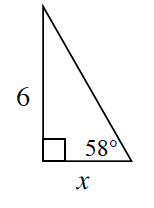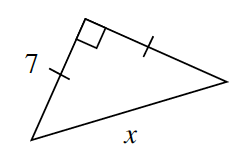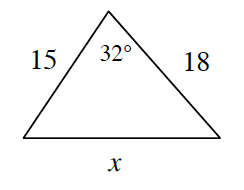### Home > CCG > Chapter 6 > Lesson 6.1.2 > Problem6-15

6-15.

Examine the triangles below. For each one, solve for $x$ and name which tool you used. Show all work.

1.Which trigonometric ratio can you use to solve for $x$?

1.What do the tick marks on the sides of the triangle mean?
How can you use that knowledge to solve for $x$?

Because of the marks, the lengths of the sides are the same.

Since this is a Right Isosceles triangle, use the Pythagorean Theorem.

$x\approx9.9$

1.Use the Law of Cosines to solve for $x$.

$x\approx9.54$